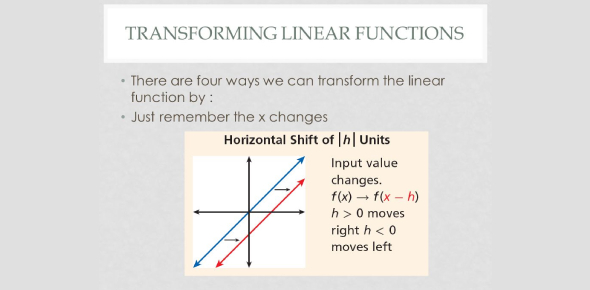Transforming Linear Functions Quiz! Test

11 Questions | Total Attempts: 453SettingsHere we bring you the Transforming Linear Functions Quiz. There are a lot of people who have a hard time when it comes to formulating linear equations, and the quiz below is designed to test out if you are among this group. All you need to do is to ensure you read the question as well as you can and choose the best suitable answer for each problem. Give it a try and keep a lookout for more questions, just like it!

• 1.
• A.

Y = 2/3x - 6

• B.

Y = -2/3x - 1

• C.

Y = -2/3x + 4

• D.

Y = 2/3x + 4

• 2.
Given a linear function with a slope of -4/3, how will the graph differ if the slope changed to 4/3?
• A.

The steepness of the line increases.

• B.

The slope of the line changes from increasing to decreasing.

• C.

The slope of the line changes from decreasing to increasing.

• D.

There is no change in the graph of the line.

• 3.
Determine the slope of the line 6x + 3y = 6.  As the x-value increases, how would you describe movement along the line?
• A.

1 unit to the right, 2 units down

• B.

1 unit to the left, 2 units down

• C.

2 units to the left, 1 unit down

• D.

2 units to the right, 1 unit up

• 4.
The line y= 2/5x + 4 is transformed to y = 4/5x + 4.  How does this new rotation affect the graph?
• A.

The slope decreases; therefore, the line is closer to the y-axis and the steepness increases

• B.

The slope decreases; therefore, the line is closer to the x-axis and the steepness decreases

• C.

The slope increases; therefore, the line is closer to the x-axis and the steepness decreases

• D.

The slope increases; therefore, the line is closer to the y-axis and the steepness increases

• 5.
• A.

The amount he charges for each job will increase by \$10.

• B.

The amount he charges for each job will decrease by \$10.

• C.

The amount he charges per hour will increase by \$10.

• D.

The amount he charges per hour will decrease by \$10.

• 6.
• A.

Y = 0.25x + 40.25

• B.

Y = 0.15x + 40.25

• C.

Y = 0.15x + 29.75

• D.

Y = 0.25x + 29.75

• 7.
• A.

The slope of the Rider truck is steeper.

• B.

The slope of the I-Haul truck is steeper.

• C.

The slope of the Budjet truck is steeper.

• D.

Cannot be determined

• 8.
• A.

The slope is steeper before the slip.

• B.

The slope is unchanged.

• C.

Cannot be determined.

• D.

There is no slope involved.

• 9.
• A.

The slope would increase.

• B.

The y-intercept would increase.

• C.

The slope and y-intercept would decrease.

• D.

The slope and y-intercept would stay the same.

• 10.
• A.

The pool company is charging more per hour.

• B.

The pool company started with a lower service call fee.

• C.

It will take less time for a customer's bill to reach \$90.

• D.

After 6 hours a customer will be billed more than \$100.

• 11.
The function f(x) = -2/3x + 2 can be formed by shifting the function y = -2/3x in which way?
• A.

Vertical shift up 2

• B.

Reflection over the x-axis

• C.

Vertical shift down 2

• D.

Horizontal shift to the left 2

Related TopicsBack to top